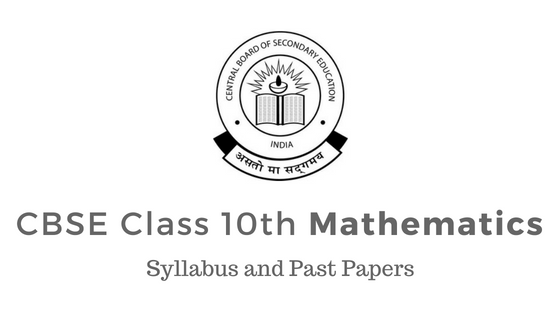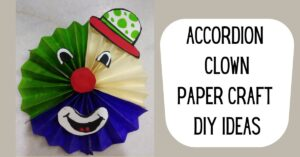# CBSE Class 10th Mathematics Syllabus 2020-21 and Past Papers (CODE NO. 041)

By SchoolMyKids Expert | 3-4 mins read | March 27, 2020CBSE Class X Mathematics (Code No. 041) Syllabus 2020-21.  Check out CBSE Class 10th Mathematics Syllabus, CBSE Class 10th Mathematics Previous year question papers, CBSE Class 10th Mathematics Sample Papers. Check out CBSE Class 10th Syllabus and Past Papers of other CBSE Class 10th Course CBSE Class 10th Science Syllabus 2020-21 and Past PapersCBSE Class 10th Mathematics Syllabus 2020-21 and Past Papers, CBSE Class 10th English Language And Literature Syllabus 2020-21 and Past Papers, CBSE Class 10th English Communicative Syllabus 2020-21 and Past Papers, CBSE Class 10th Social Science Syllabus 2020-21 and Past Papers or check out CBSE Class 10th Previous Year Question Papers and Sample Papers Solved. Continue to read for CBSE Class 10th Syllabus Mathematics.

## Section - Wise Weightage - CBSE Class 10th Mathematics

 Units Unit Name Marks I NUMBER SYSTEMS 06 II ALGEBRA 20 III COORDINATE GEOMETRY 06 IV GEOMETRY 15 V TRIGONOMETRY 12 VI MENSURATION 10 VII STATISTICS & PROBABILTY 11 Total 80

#### UNIT I: NUMBER SYSTEMS

1. REAL NUMBERS                                                                        (15 Periods)

Euclid’s division lemma, Fundamental Theorem of Arithmetic - statements after reviewing work done earlier and after illustrating and motivating through examples, Proofs of irrationality of  2,  3,  5 Decimal representation of rational numbers in terms of terminating/non-terminating recurring decimals.

#### UNIT II: ALGEBRA

POLYNOMIALS                                                                              (7 Periods)

Zeros of a polynomial. Relationship between zeros and coefficients of quadratic polynomials. Statement and simple problems on division algorithm for polynomials with real coefficients.

PAIR OF LINEAR EQUATIONS IN TWO VARIABLES                     (15 Periods)

Pair of linear equations in two variables and graphical method of their solution, consistency/inconsistency.

Algebraic conditions for number of solutions. Solution of a pair of linear equations in two variables algebraically - by substitution, by elimination and by cross multiplication method. Simple situational problems. Simple problems on equations reducible to linear equations.

Standard form of a quadratic equation ax2 + bx + c = 0, (a ≠ 0). Solutions of quadratic equations (only real roots) by factorization, by completing the square and by using quadratic formula. Relationship between discriminant and nature of roots.

Situational problems based on quadratic equations related to day to day activities to be incorporated.

ARITHMETIC PROGRESSIONS                                                      (8 Periods)

Motivation for studying Arithmetic Progression Derivation of the nth term and sum of the first n terms of A.P. and their application in solving daily life problems.

#### UNIT III: COORDINATE GEOMETRY

LINES (In two-dimensions)                                                               (14) Periods

Review: Concepts of coordinate geometry, graphs of linear equations. Distance formula. Section formula (internal division). Area of a triangle.

#### UNIT IV: GEOMETRY

TRIANGLES                                                                                  (15) Periods

Definitions, examples, counter examples of similar triangles.

1. (Prove) If a line is drawn parallel to one side of a triangle to intersect the other two sides in distinct points, the other two sides are divided in the same ratio.
2. (Motivate) If a line divides two sides of a triangle in the same ratio, the line is parallel to the third side.
3. (Motivate) If in two triangles, the corresponding angles are equal, their corresponding sides are proportional and the triangles are similar.
4. (Motivate) If the corresponding sides of two triangles are proportional, their corresponding angles are equal and the two triangles are similar.
5. (Motivate) If one angle of a triangle is equal to one angle of another triangle and the sides including these angles are proportional, the two triangles are similar.
6. (Motivate) If a perpendicular is drawn from the vertex of the right angle of a right triangle to the hypotenuse, the triangles on each side of the perpendicular are similar to the whole triangle and to each other.
7. (Prove) The ratio of the areas of two similar triangles is equal to the ratio of the squares of their corresponding sides.
8. (Prove) In a right triangle, the square on the hypotenuse is equal to the sum of the squares on the other two sides.
9. (Prove) In a triangle, if the square on one side is equal to sum of the squares on the other two sides, the angles opposite to the first side is a right angle.

CIRCLES                                                                                   (8) Periods

Tangent to a circle at, point of contact

1. (Prove) The tangent at any point of a circle is perpendicular to the radius through the point of contact.
2. (Prove) The lengths of tangents drawn from an external point to a circle are equal.

CONSTRUCTIONS                                                                        (8) Periods

1. Division of a line segment in a given ratio (internally).
2. Tangents to a circle from a point outside it.
3. Construction of a triangle similar to a given triangle.

#### UNIT V: TRIGONOMETRY

INTRODUCTION TO TRIGONOMETRY                                        (10) Periods

Trigonometric ratios of an acute angle of a right-angled triangle. Proof of their existence (well defined); motivate the ratios whichever are defined at 0O and 90O. Values (with proofs) of the trigonometric ratios of 30O, 45O and 60O. Relationships between the ratios.

TRIGONOMETRIC IDENTITIES                                                      (15) Periods

Proof and applications of the identity sin2A + cos2A = 1. Only simple identities to be given. Trigonometric ratios of complementary angles.

HEIGHTS AND DISTANCES:                      (8) Periods

Angle of elevation, Angle of Depression. Simple problems on heights and distances. Problems should not involve more than two right triangles. Angles of elevation / depression should be only 30O, 45O, 60O.

#### UNIT VI: MENSURATION

AREAS RELATED TO CIRCLES                                                     (12) Periods

Motivate the area of a circle; area of sectors and segments of a circle. Problems based on areas and perimeter / circumference of the above said plane figures. (In calculating area of segment of a circle, problems should be restricted to central angle of 60°, 90° and 120° only. Plane figures involving triangles, simple quadrilaterals and circle should be taken.)

SURFACE AREAS AND VOLUMES                                                 (12) Periods

1. Surface areas and volumes of combinations of any two of the following: cubes, cuboids, spheres, hemispheres and right circular cylinders/cones. Frustum of a cone.
2. Problems involving converting one type of metallic solid into another and other mixed problems. (Problems with combination of not more than two different solids be taken).

#### UNIT VII: STATISTICS AND PROBABILITY

STATISTICS                                                                                   (18) Periods

Mean, median and mode of grouped data (bimodal situation to be avoided). Cumulative frequency graph.

PROBABILITY                                                                          (10) Periods

Classical definition of probability. Simple problems on single events (not using set notation).

## CBSE Class 10th Mathematics Prescribed Books

1. Mathematics - Textbook for class IX - NCERT Publication
2. Mathematics - Textbook for class X - NCERT Publication
3. Guidelines for Mathematics Laboratory in Schools, class IX - CBSE Publication
4. Guidelines for Mathematics Laboratory in Schools, class X - CBSE Publication
5. Laboratory Manual - Mathematics, secondary stage - NCERT Publication
6. Mathematics exemplar problems for class IX, NCERT publication.
7. Mathematics exemplar problems for class X, NCERT publication.

## CBSE Class X Mathematics Question Paper Format and Design

Mathematics (Code No. 041) board examination duration is 3 hrs and is of Marks: 80 .

 S. No. Typology of Questions Very Short Answer (VSA) (1 Mark) Short Answer -I (SA) (2 Marks) Short Answer -II (SA) (3 Marks) Long Answer (LA) (4 Marks) Total Marks % Weightage (approx.) 1 Remembering (Knowledge based- Simple recall questions, to know specific facts, terms, concepts, principles or theories; Identify, define, or recite, information) 2 2 2 2 20 25% 2 Understanding (Comprehension- to be familiar with meaning and to understand conceptually, interpret, compare, contrast, explain, paraphrase, or interpret information) 2 1 1 4 23 29% 3 Application (Use abstract information in concrete situation, to apply knowledge to new situation; Use given content to interpret a situation, provide an example, or solve a problem) 2 2 3 1 19 24% 4 Higher Order Thinking Skills (Analysis & Synthesis- Classify, compare, contrast, or differentiate between different pieces of information; Organize and /or integrate unique pieces of information from  variety of sources ) - 1 4 - 14 17% 5 Evaluation ( Judge, and/or justify the value or worth of a decision or outcome, or to predict outcomes based on values) - - - 1 4 5% Total 6x1=6 6x2 =12 10x3 =30 8x4 =32 80 100%

Note: One of the LA will be to assess the values inherent in the texts.

INTERNAL ASSESSMENT                                                                            20 Marks

• Periodical Test                                                                       10 Marks
• Note Book Submission                                                      05 Marks
• Lab Practical (Lab activities to be done from the prescribed books) 05 Marks

#### Categories

Education CBSE Board Boards
SchoolMyKids provides Parenting Tips & Advice to parents, Information about Schools near you and School Reviews, . Use SchoolMyKids Baby Names Finder to find perfect name for your baby.
##### SchoolMyKids Expert

Last Updated: March 27, 2020
This disclaimer informs readers that the views, thoughts, and opinions expressed in the above blog/article text are the personal views of the author, and not necessarily reflect the views of SchoolMyKids. Any omission or errors are the author's and we do not assume any liability or responsibility for them.

#### Recent Posts